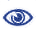# The Prague Stringology Conference 2008

Average Value of Sum of Exponents of Runs in Strings

 Abstract: A substring w[i..j] in w is called a repetition of period p if s[k] = s[k+p] for any i ≤ k ≤ j - p. Especially, a maximal repetition, which cannot be extended neither to left nor to right, is called a run. The ratio of the length of the run to its period, i.e. (j - i+1)/p, is called an exponent. The sum of exponents of runs in a string is of interest. The maximal value of the sum is still unknown, and the current upper bound is 2.9n given by Crochemore and Ilie, where n is the length of a string. In this paper we show a closed formula which exactly expresses the average value of it for any n and any alphabet size, and the limit of this value per unit length as n approaches infinity. For binary strings, the limit value is approximately 1.13103.

 Download paper:PostScript PDF BibTeX reference
 Download presentation: